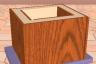http://people.csail.mit.edu/jaffer/Work/thermal

# Scheme for Thermal Simulation

For systems with a few time constants, spreadsheet programs can provide quick and easy simulation of heat and temperature over short durations. As systems grow more complex or if simulation of a typical meteorological year is required, spreadsheets become unwieldy and brittle.

Scheme for Linear System Analysis shows how to solve linear systems in the frequency domain; this page details how to solve them in the time domain.

Lumped element thermal simulations can be modeled as electrical networks composed of grounded capacitors (thermal mass) and resistors (thermal resistance). With all their poles lying in the left half-plane, such systems are unconditionally stable; and quantization errors are attenuated by the integrations associated with the capacitors.

## Switched Capacitors

Each resistor can be modeled as a switched-capacitor operating at the sampling frequency fc = 1 / tc.

Resistor (in Circuit)Equivalent Switched-Capacitor
```Ei       Ri,k      Ek
o---+---/\/\---+---o
| >-Ii,k-> |
|          |
_|_        _|_
Ci  ---        --- Ck
|          |
_|_        _|_
///        ///
```
```  _
/ \_/ >--+------+
fc      |   ___|___
___O___ |   |_
Ei      |   |  |     |    Ek
o---+--+   +--+-||--+-+---o
| >-Ii,k->  Ci,k  |
|                 |
_|_               _|_
Ci  ---               --- Ck
|                 |
_|_               _|_
///               ///
```
Ii,k = (Ek - Ei) / Ri,k Ii,k = (Ek - Ei) · Ci,k · fc

 Equating the Ii,k currents at sampling frequency fc gives: Ci,k = 1Ri,k · fc = tc · Gi,k

## Simulating

This technique requires storage for (scalars) charge Qi and voltage Ei for each (capacitor) node.

• Calculate each node's voltage from its charge and capacitance: Ei = Qi / Ci
• For each pair of connected nodes indexed by i and k, where i < k:
• calculate the transfer charge Qt = (Ek - Ei) · Ci,k = (Ek - Ei) · tc · Gi,k
• add Qt to Qi (the charge accumulating in Ci)
• subtract Qt from Qk (the charge accumulating in Ck)
• Repeat this procedure T · fc times, where T is the desired length of the simulation in seconds.